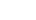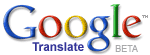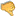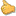ترجم الموضوع الى العربية# The Mathematical Equation of RealityHassan Ajami
2019 / 11 / 9

We could successfully mathematize reality in the following way: reality = abstract x concrete. This mathematical equation analyzes reality in terms of the abstract and the concrete, such that reality is equal to the abstract multiplied by the concrete. And it could be abbreviated as r = a x c (such that r is reality, while a is the abstract, and c is the concrete).

The mathematical equation of reality possesses a successful explanatory power, leading to its acceptance. For example, since reality = abstract x concrete, it follows that reality (i.e. the universe) is abstract, such as being a set of abstract information (as the physicist John Wheeler says),�-or-such as being abstract mathematical equations (as the physicist Max Tegmark maintains). This shows that the mathematical equation of reality is successful in explaining why the scientists are successfully able to explain reality and/or the universe as being abstract information�-or-as being abstract mathematical equations. And hence, the mathematical equation of reality gains a successful explanatory power.

Further, since reality = abstract x concrete, it follows that reality and/or the universe is also concrete, such as consisting of material atoms and/or material subatomic particles, as many physicists say. This shows that the mathematical equation of reality successfully explains why scientists are successful in explaining the universe as being concrete, such as consisting of material atoms. Thus, the mathematical equation of reality gains this additional explanatory power.

In other words, reality could be successfully explained as being abstract and also as being concrete because reality = abstract x concrete. If reality is not equal to the abstract multiplied by the concrete, then it will not be the case that we can successfully explain reality as abstract and concrete at the same time. Yet we can successfully explain reality as being abstract and concrete at the same time (as different scientists do). Therefore, reality is equal to the abstract multiplied by the concrete.

In addition, the mathematical equation of reality resolves a fundamental conflict in philosophy, namely the conflict between idealism and materialism. For idealism, the universe is mental and/or mentally dependent, while, for materialism, the universe is material and everything is reducible to and/or dependent on matter. But, according to the mathematical equation of reality, reality = abstract x concrete. And therefore, reality is abstract, such as being mental as the idealists hold, and reality is also concrete, such as being material as the materialists maintain. Hence, from the perspective of the mathematical equation of reality, both idealism and materialism are true, leading to the resolution of the conflict between idealism and materialism. And this is a virtue which speaks for the acceptance of the mathematical equation of reality.

It is plausible to conceive reality as both abstract (i.e. ideal) and concrete (i.e. material) because reality itself consists of diverse levels. In other words, there are different levels of reality, such that at one level, reality is abstract and hence ideal, while, at another level of reality, reality is concrete and hence material. If there weren�t different levels of reality, then it would have been impossible to successfully describe the same reality in idealistic terms, such as describing reality as consisting of abstract information (as some scientists do), and to describe the same reality at the same time in materialistic terms, such as consisting of material atoms (as other scientists do).

The mathematical equation of reality has some philosophically interesting implications, such as implying that there are different realities and/or universes. If the equation r = a x c is true, then r divided by a is equal to c, and r divided by c is equal to a, and obviously r = a x c. Thus, there are diverse realities and/or universes, such as realities�-or-universes where their concrete entities are maximized while their abstractness is minimized (corresponding to r divided by a is equal to c), and universes where their abstractness is maximized while their concreteness is minimized (corresponding to r divided by c is equal to a), in addition to universes�-or-realities where their abstractness and concreteness are maximized together�-or-minimized together (corresponding to r = a x c). Another interesting conclusion of this equation is that reality is a matter of degree. Since r = a x c, it follows that when a and c are maximized, reality will be maximized, while when a and c are minimized, reality will be minimized. Hence, reality is a matter of degree.

Reality is abstract and concrete. This is why we could successfully describe reality as being abstract and as being concrete at the same time, exactly as the different scientific theories do. And this is also why both idealism and materialism are true, leading to resolve a basic philosophical debate between the idealists and the materialists.Bad 12345678910 Very good